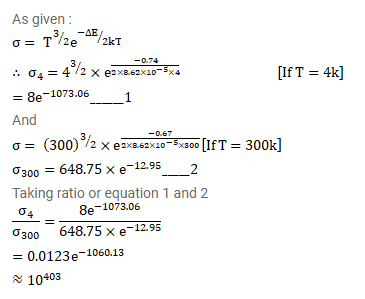# The conductivity of a pure semiconductor is roughly proportional to

Question:

The conductivity of a pure semiconductor is roughly proportional to $\mathrm{T}^{3 / 2} \mathrm{e}^{-\Delta \mathrm{E} / 2 \mathrm{kT}}$ where $\Delta \mathrm{E}_{\text {is }}$ the band gap. The band gap for germanium is $0.74 \mathrm{eV}$ at $4 \mathrm{k}$ and $0.67 \mathrm{eV}$ at $300 \mathrm{k}$. By what factor does the conductivity of pure germanium increase as the temperature is raised from $4 \mathrm{~K}$ to $300 \mathrm{~K}$ ?

Solution: